Search IntMath
Close

450+ Math Lessons written by Math Professors and Teachers

5 Million+ Students Helped Each Year

1200+ Articles Written by Math Educators and Enthusiasts

Simplifying and Teaching Math for Over 23 Years

# Modeling fish stocks

By Murray Bourne, 28 May 2012

When I was a kid, fish was cheap (because there was plenty of it) and chicken was expensive (because factory-style chicken farming was not so widespread).

In more recent years, fish has been getting more expensive because it's getting harder to find. And of course, this is due to overfishing and insufficient aquaculture.

Fish are one of the best protein sources we have, especially as they provide us with Omega 3 fatty acids (which are essential for brain development), and they can improve cholesterol.

## Collapsing fish stocks

There have been some well-documented collapses in fish stocks, including:

1. Atlantic cod, which went from a sustainable rate of 250,000 tonnes per year in the late 1950s, to 800,000 tonnes in 1968, and then collapsed to 1,700 tonnes by 1995.
2. Norwegian herring, which collapsed in the late 1960s and the fishery was closed from 1977 to 1981.
3. Peruvian anchovy, which collapsed in the 1970s, especially due to overfishing during El Niño events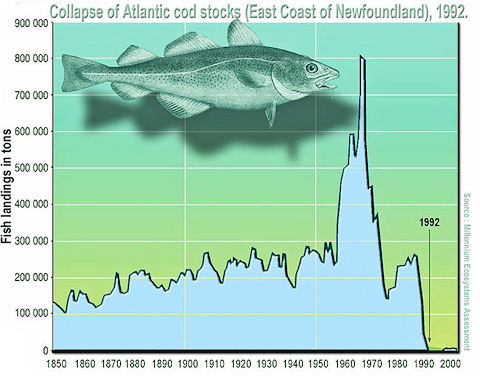[Image source]

## Mathematical modeling for better fish management

It is possible to have a sustainable fishing industry with proper planning and strictly enforced quotas.

One way to achieve good planning is to model the inputs (food availability, breeding rate, water temperature, pollutants) and the outputs (natural deaths, commercial fishing). Such modeling can be done using differential equations.

Differential equations involve an instantaneous time rate of change term,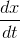, where x is the amount of fish in the sea (as a mass, or possibly as a gross number of fish), and t is the time (usually in months or years).

Built in to each fish stock equation is a positive (growth) term (dependent on food supply, breeding rates, etc), and a negative (inhibition) term (due to limits on food availability, etc).

Here's a highly simplified example of a model for the amount of fish expected in a particular area bounded by some fixed geographic boundary (for example, a bay):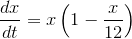The growth term is the x outside the brackets on the right hand side, and the negative inhibition term is contained in the brackets. The number "12" is important, since this indicates a stable value for the amount of fish, all else being equal.

We need a starting value. In this example, for illustration, we start with 500 tonnes of fish in the sea, and we write it like this (our unit for mass of fish throughout the equations and graphs in this discussion is "thousand tonnes"):

x(0) = 0.5

Next, we solve our differential equation, with the given initial condition. (I have used computer software to solve it. For those interested to see how it is done on paper, see the Comments for this post below, and Solving DEs by Separation of Variables).

Solution: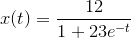Our solution is a function of time (which will be in years for the purpose of this discussion), and we can graph it to see what is going on.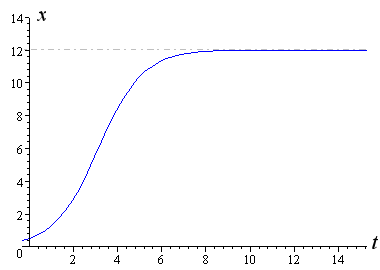Notice at t = 0, the value of x is 0.5, which is what we specified earlier. Notice also that the quantity of fish grows exponentially at first (for about 3 years), then grows less rapidly, and eventually levels off after about 9 years at the stable 12,000 tonne value.

In practical terms, this means there is enough food, and other conditions are just right, for the fish to maintain a mass of around 12,000 tonnes in our particular bay.

(Many populations follow this kind of S-shaped growth, called "logistic" growth.)

## Overpopulated case

Let's now look at the case where, for some reason (it could be a temporary increase in food supply), the amount of fish went beyond 12,000 tonnes. If the food supply then returns to normal, the bay will not be able to sustain so many fish.

To illustrate, we let the initial condition be 14,000 tonnes of fish.

x(0) = 14

This time when we solve the differential equation, we get this expression: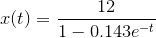Now for the graph: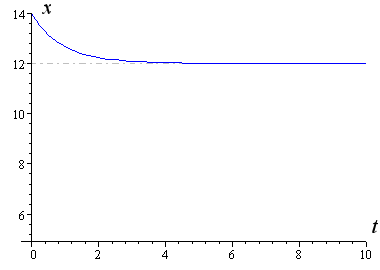We see the amount of fish drops down to the stable value of 12,000 tonnes. (As t gets very large, the denominator of our fraction approaches 1, hence the value of the fraction approaches 12.)

## Sustainable fishing case

Next, let's assume a fishing trawler moves into the bay. They take out 2,000 tonnes of fish per year. (It's a small trawler. Typically trawlers can catch 4,000 to 5,000 tonnes per year).

Our differential equation becomes: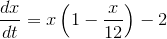We choose our starting value as the stable value of 12,000 tonnes.

x(0) = 12

Here is the graph: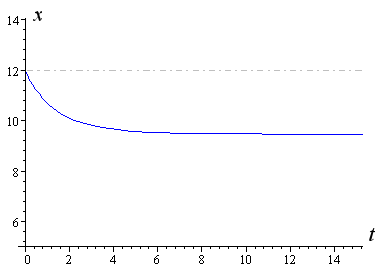We see that fishing has dropped the overall amount of fish (by slightly more than the 2,000 tonnes fished each year), but fishing stocks are stable.

(I haven't included the solution for x in this case, since it involves the real part of the hyperbolic tanh of a logarithm, which is somewhat beyond this discussion.)

## Unsustainable fishing case 1

In our first example above, we started with a small amount of fish (500 tonnes) and plenty of food.

If we try to fish 2,000 tonnes per year, of course our fish stocks will collapse.

x(0) = 0.5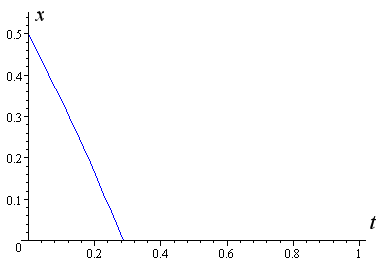There are not enough adult fish to lay eggs, so no new fish are being produced, and the result is the numbers reduce rapidly to zero.

## Unsustainable fishing case 2

This time, let's start with a very robust amount of fish (20,000 tonnes) and use a fishing rate of 6,000 tonnes per year.

This is the differential equation for this situation, and the initial value.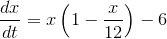x(0) = 20

The solution in this case is given by: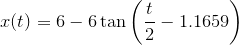Here's the graph: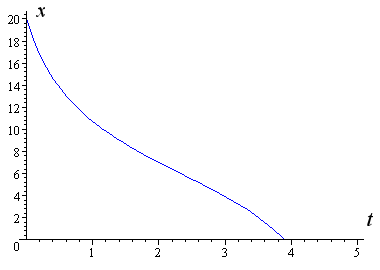The collabse is not quite so abrupt, but it's still a collapse. This fishery cannot sustain this rate of fishing, even though we started with a highly overpopulated situation.

## El Niño effect

The Pacific El Niño occurs every 5 years or so. In normal years, cold water upwells along the coast of Peru, bringing with it an explosion of sea life. The Peruvian anchovy does very well in such conditions. The anchovy is very important for Peru's economy, and the fishmeal industry provides protein for a wide range of industries.

However, in El Niño years, the Peruvian waters become very warm, the amount of food for the anchovies drops dramatically, and so the anchovy fish catch drops.

The following is a highly simplified model that takes into account El Niño effects.

We assume the El Niño occurs regularly every 5 years. We start with a high fish stock rate (20 tonnes) to see more clearly what happens.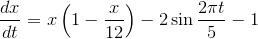x(0) = 20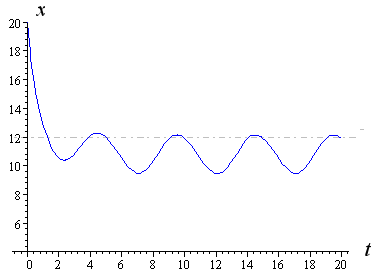We can see there are troughs at year 2, 7, 12 and 17. These are El Niño years, when the amount of food available drops, and so the fish stocks also drop. In the intermediate years, 5, 10, 15 and 20, conditions return to normal and we see a good recovery in fish stocks.

In practice, the drop in fish numbers is quite sudden, and the build up is a lot slower than indicated by the regular curves above.

(It is not possible to solve this differential equation using normal algebraic methods. I needed to solve it numerically, and the software gave me a set of numbers which I used to give the above graph.)

## Conclusion

During the 21st century, we can expect to see more fish stock collapses. It is inevitable with the increase in human population, the rapid rise of the middle class in China and India (and increasingly, Africa), overfishing, pollution and global warming. As land-based protein production becomes difficult due to polluted water, depleted topsoil and yes, global warming, there will be even more pressure on our ocean animals.

So whould we stop eating fish? I hope not. But at least we should be more active in finding out where the fish comes from, and only buy fish from companies that use sustainable practices. Look for the Marine Stewardship Council logo on the packaging.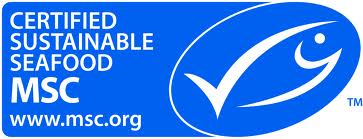Logo of the Marine Stewardship Council

There are opportunities for those with math skills in all this. Learn as much as you can about mathematical modeling, and get involved in planning for more sustainable fishing, especially in your local area.

A billion people will benefit from your skills.

This recent TED talk describes a census of the oceans, and several warnings about the future.

### 17 Comments on “Modeling fish stocks”

1. Mike London says:

Very interesting simplified explanation in the use of a sigmoidal (logistic) curve. Has work been done using the Gompertz curve?

2. ray says:

Hi Murray,

Liked the post, got the concept.

But...
I'm stuck
at the "step X step mechanics" of
going/deriving from each:
dx/dt equation.
to the corresponding:
x(t) equation
in the post.

Also tried Wolfram Alpha Calculator,
etc, with no luck.

Anyway you can add the instructions "step x step" ,
or calculator instructions
(for at leastthe first dx/dt to x(t) example ?).

Thanks Murray! - really appreciate your blog.
Ray
SF

3. Murray says:

Most articles on mathematical modelling get totally bogged down in the mechanics of the algebra, and these days with computer-based math tools, that's rapidly becoming less important.

But since you asked :-), here's an outline of how to do the first one in the article.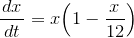Separate variables: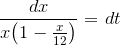Multiply top and bottom of left side by 12 to make life easier: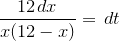We next need to integrate. The right hand side simply gives "t".

We need to use partial fractions for the left hand side: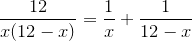Now integrate (assume all logarithm functions have the requisite absolute value signs):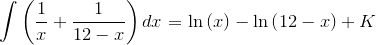Tidying up and putting it together with our integral of dt gives: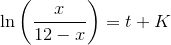Find K using the initial condition x(0) = 0.5, and some logarithm magic: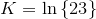So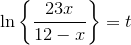Next, find "e to the power of both sides":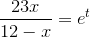Next, perform a bunch of algebra to get x by itself, and get: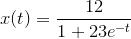Hope it makes sense.

Here is Wolfram|Alpha's solution

4. Murray says:

Hi Mike. Yes, the Gompertz function would also do the trick for some of these models, since it is derived from the logistic equation.

5. ray says:

Thanks for the precise steps, Murray.

Whew!
No wonder I couldn't derive the steps myself,
(fish brain on-board, here...).

Thanks again!
Ray
SF

6. ray says:

OK!

Thanks! It works.
(I will also dutifully

Thanks !!
Ray
SF

7. Mike London says:

Thank you Murray, there are so many more applications of these little used distributions.

Unfortunately some new graduates think that we live in a Gaussian world and adjust their data to conform to a normal distribution.

8. Mathblogging.org Weekly Picks « Mathblogging.org — the Blog says:

[...] squareCircleZ, Murray Borne models fish [...]

9. 87th Carnival of Mathematics | Random Walks says:

[...] Bourne submitted this awesome post about modeling fish stocks. Murray says his post is an “attempt to make mathematical modeling a bit less scary than in [...]

10. alQpr » Blog Archive » Sustainable Yield? says:

[...] Bourne at ‘squareCircleZ’ has a great post on modeling fish stocks as an interesting applied example for a math [...]

Hi! I think it is a good idea to make mathematics more practical more especially using modeling to solve real world problems

12. anonymous says:

Found a typo: x(t) for the overpopulated case should have a minus sign in the denominator. Can't get x(0)=14 otherwise.

13. Murray says:

@anon: Thanks! You were right - it should have been a minus. It's fixed now.

14. anonymous says:

The Preview robo-editor appears to paraphrase what I write and fails badly at it. It posted "K less than or equal to 3 is sustainable. K greater than 3 is fatal" as "K3 is fatal" so I had to replace symbols with words. Quite frustrating.

The graph for the sustainable fishing case (dx/dt=x*(1-x/12)-2, x(0)=12) asymptotes to x(∞) ≈ 9.5 (by eyeball) which suggests that x(∞) can be solved for by setting dx/dt=0 for the general case:

dx/dt = x * (1 - x/12) - K = 0 where K = harvest rate

x^2 - 12x + 12K = 0

x(∞) = [12 +/- (12^2 - 4*12K)^0.5] / 2 = 6 +/- (36 - 12K)^0.5

For K=2, x(∞) = 6 +/- 12^0.5 = 9.464 or 2.536 which agrees well with the graph. The smaller root does not work because nearby x around 2.536 does not converge to but diverges away from it.

Sustainable Ks exist only when real roots exist for x(∞), i.e., when (36 - 12K)>=0. K less than or equal to 3 is sustainable. K greater than 3 is fatal. x(∞)=6 for K=3 when the square root term vanishes.

Another way to get the same result is to look for maximum growth in x which translates into the maximum sustainable harvest. We set d(dx/dt)/dx=0 and we get 1-x/6=0 or x=6 which corresponds to dx/dt=6*(1-6/12)=3.

The El Nino sinusoid on the graph fluctuates about x(∞) ≈ 10.8 (by eyeball) which agrees closely (enough) with x(∞)=6 +/- 24^0.5=10.899 or 1.101 for the K=1 case.

15. Murray says:

Actually, the "robo-editor" behaved quite correctly. The Comment box allows users to include some HTML. When entering math, we need to be careful about the "<" (less than" sign, since it indicates the beginning of an HTML tag, and the ">" sign is the end of the tag. Such tags do not appear on the page, since they are meant to tell the browser what to do.

So in your case, “K < or equal to 3 is sustainable. K > 3 is fatal” will certainly come out as “K3 is fatal”.

The trick is to use "&lt;" when you want a less than sign, and "&gt;" when you want a greater than sign.

Ahh, the joys of math on the Web.

16. anonymous says:

@Murray: Thanks for the enlightenment. It looks like the editor doesn't display infinity and approximately-equal-to symbols either. x(infinity) approximately equals to 9.5 posted as "x(?)?9.5"

17. Murray says:

@anonymous: OK, I've fixed all those infinities and approximately equal to's.

FYI, "&infin;" will give you the "infinity" symbol, and "&asymp;" will give you "approximately equal to" symbol.

### Comment Preview

HTML: You can use simple tags like <b>, <a href="...">, etc.

To enter math, you can can either:

1. Use simple calculator-like input in the following format (surround your math in backticks, or qq on tablet or phone):
a^2 = sqrt(b^2 + c^2)
(See more on ASCIIMath syntax); or
2. Use simple LaTeX in the following format. Surround your math with $$ and $$.
$$\int g dx = \sqrt{\frac{a}{b}}$$
(This is standard simple LaTeX.)

NOTE: You can mix both types of math entry in your comment.